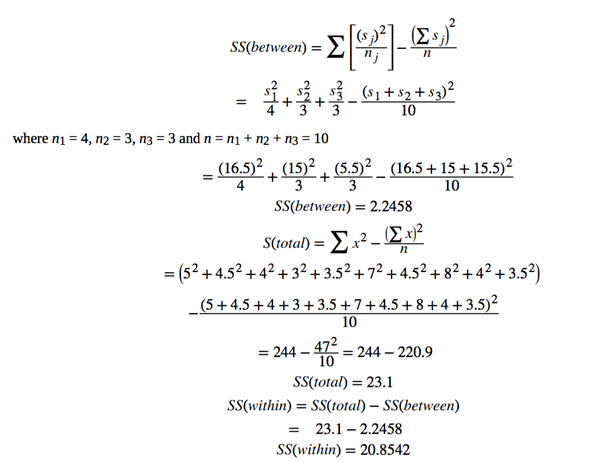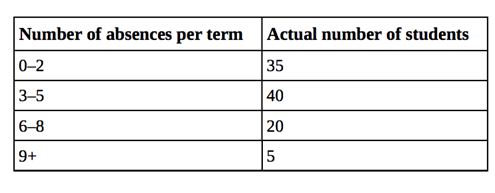# F-distribution Assignment Help

## F-distribution assignment help / Chi-square & one-way Anova

In various fields of social sciences, economics, business management etc. use statistical measures which have plenty of categories and groups. For example, a customer will compare the quality of several refrigerators before buy one. An environmentalist will want to know how the carbon footprint varies between different districts of the same city. For simplicity and differentiation, statisticians have invented a way called ANOVA to conduct hypotheses for comparing two or more categories. To estimate values of certain parameters we need to used confidence intervals. Single way Anova is the fundamental and the easiest form of ANOVA. F distribution is a method used for conducting an ANOVA about which we will discuss below.

First, to understand how the data is organised we need to understand what we mean by confidence intervals as a measure to estimate data:

## Confidence Intervals

In inferential statistics, “Confidence Intervals” is the first and one of the most important topics.

For the purpose of estimating mean (µ) which is a population parameter, in Inferential Statistics, we require some sample data.  There are different ways to estimate value of parameters such as point estimates which uses limited, random samples. This is the reason why the estimates are nearly always wrong.

## Understanding Confidence Interval

To solve this problem of being nearly always wrong, we quantify our margin of error to determine how wrong we may be. We add or subtract this calculation of margin of error from our point estimates to form a Confidence Interval (CI)

The official definition is : “A confidence interval gives an estimated range of values which is likely to include an unknown population parameter, the estimated range being calculated from a given set of sample data.” (Valerie J. Easton and John H. McColl, 2012)

CI= point estimate ± margin of error

Confidence Intervals are mentioned in tables and graphs, hand in hand with point estimates of the same parameters, depiction the deviations in estimates.

### The Point Estimate

In simple words, the best assumption of the value of a population parameter, in this case mean is called the point estimate.

Example:Note: Even though it is our best assumption, we need to leave room for some error as it is only an assumption. Probably, why point estimates go hand in hand with margins of these errors.

### Margin of Error

This depicts our best guess of an amount by which we believe, the point estimates are wrong or in error. In simple terms, the difference between the actual estimate from the point estimate by a desired “level of confidence” is called the margin of error.

### Interpreting the Confidence Intervals

While constructing a confidence interval, we assume that it will lead to an unknown estimation of the parameter. Basically, at some level of confidence, plausible values if of the parameter are called Confidence Intervals. The possibility that the CI constructed using random sample will lead to the actual parameter is calculated by the % chance. For example, if we estimate a Confidence Interval of 90%, it means that 90% of the times, this type of CI will estimate the actual and true parameter value. However, we must also note, that there is still room for error as we may never know if they really did capture the true value.

Formula

It can be seen with the formula:

CI for unknown parameter = point estimate ± margin of error

Margin Error can also be referred as ME for convenience.

Margin of error (ME)

ME = (critical value) * (standard error of point estimate)

CI = point estimate ± (critical value) * (standard error)

To construct a Confidence Interval for a population mean, we need to clarify the following: The Point estimate = sample mean,

Critical Value = calculated with reference to t-distribution,

Standard error = standard deviation of the sample / square root of the size of the

We also need to keep in mind that “t” is referred to the critical value, to be found under the t-table in the column for the respective interval.

The idea behind CI was constructed to give us a plausible way to deal with the uncertainty that arises out of the subset of population that is randomly selected. The errors are so inherent that for years, valuators couldn’t figure out the correct estimation techniques barring the uncertainties. They were later again answered by Bayesian Inference by leading to the discovery of Credible Intervals. The idea of calculating uncertainties in an estimate, in itself being dichotomous, with the help of intervals is not just a mathematical problem but also a philosophical one.

#### One- Way ANOVA

Now that we’ve understood what Confidence intervals stand for we can carry on with comprehending ANOVA which is considered to be a breakthrough in analysing of relationships.

The full form of ANOVA is “Analysis of Variance”. It is used to test the different between different groups and their means. It uses variance to help us find out if the means are similar. There are couple assumptions are needing to be completed before we conduct the one-way ANOVA test.

1. Each variable from a sample needs to be normally distributed.
2. The population have equal variances (or standard deviations).
3. They are all independent and randomly selected.

### The Alternative and Null Hypotheses

The alternative hypothesis state that there has to be at least one mean of a group which is not the same and is different. Null hypothesis basically means that the means of the entire population is the same. An example can be seen below:

Example:

If there are k groups:

H0: μ1 = μ2 = μ3 = ... = μk

Ha: this refers to at least two means in the group which are μ1, μ2, μ3, ..., μk are not equal.

This can be depicted in a graph. Here distribution of values are represented on box portrayed through the horizontal line across them. This will help us understand the hypothesis test.The green box plots show that if the null hypothesis is not true, due to different means, the variance of the data combines is bigger.The red box plots show that , in this case if he null hypothesis is true ,H0: μ1 = μ2 = μ3 along with the other three populations have the same distribution. The variance of individual population group is almost the same as the variance of the data combined.

### The F Distribution

The form of distribution that is uses to conduct the hypothesis test is called the F distribution. An English statistician by the name of Ronald Fisher invented it, hence it was named after him.

The distribution gives us the F- ratio that consists of two sets for the numerator and denominator with varying degrees of freedom.

For the purpose of calculating the F ratio, we have to make certain estimates for the variance :

Variance between samples:  N is multiplied by the variance of the means gives us  σ² . The covariance has to be weighted in cases where the sample sizes are different.  This is also called explained variation.

Variance within samples: Average of all the variances when pooled is shown again by σ². Same way, variances are weighted when samples sizes are different. This is called unexplained variation.

Sum of squares is used to show the variation among the samples = SS between

Sum of squares that is used to show variations within the samples = AA within

Addition of weight squared quantities gives us the “sum of squares”.

The same way MS is the mean square and will be denoted by MSwithin and MSbetween

This only works if MSbetween can be affected by the differences in means of the population and their groups. Only then is the ANOVA test successful.

The F-ratio can be given by :

F = MSbetween / MSwithin

If both prove the null hypothesis true that they give the same vale, then F ratio should be 1. The variations will only occur due to sampling errors. MSbetween is basically the addition of variances of difference between samples and the total population variance. MSwithin is just the variance of the population. If in  a case the null hypothesis is not considered try, MS between will come out to be greater than MSwithin which we give a larger F ratio.

When groups are the same size, F-ratio can be show by the below mentioned formulas.Using SPSS for simplification, the data is formed in a table. One-Way ANOVA results are often displayed in the same way as the table below.Example: Various Diets are to be tested to calculate the weigh loss mean. The table depicts the weight losses as resulted by ANOVA:We’ll require the following estimations for the ANOVA table to be complete so we can conduct the hypothesis test.The bigger of the F values are placed on the right tail of curve of F distribution, hence t is always right tailed. This is reason why the H0 is rejected and results in false.

Some properties of the F distribution are given below:

1. The F curve is skewed to the right and is not symmetrical
2. If the numerators and denominators with varied degrees of freedom get larger, the normal is approximated by the curve.
3. The F ratio is > / = 0 .

The curve is depicted below in the figures with varying degrees of freedom!## Chi- square Distribution

In inferential Statistics, Chi- square is one of the most widely used probability distribution. It is fundamentally used to test hypotheses.

It is considered to be part of the definition of f-distribution, ANOVA and regression. The main reason why it’s used in hypothesis testing is due its similarity and relationship with normal distribution.

It can be depicted through the following notation:

χ ∼ χd²f

in which df depicts degree of freedom. Chi- square is used depending on this.

Here, for the respective distribution the mean is df and the standard deviation is σ = sq. root of 2(d f) .

#### Goodness-of-fit Test

This particular type of hypothesis testing involves us determining if the data set collected I “fit” for this distribution or not. To test the same we used the following formula :where : the observed value are depicted via O, the expected values are depicted via E and the total number of categories and data cells are shown by k. here the fit test reveals that it is always right tailed as well. If the E and O are far away from each other and will lead to it being way out of the right tail. This can be shown in the example below:

Example: A large number of pupils are not attending classes for Accounting, which the professors have observed is directly related to them dropping out of them. A study is conducted assuming perception of student absenteeism which results in the following table:An actual survey was then done to determine the actual absences in Accounting class showing the following results:Here to understand the alternative and null hypotheses, we do a goodness of fit test.

H0 depicts that faculty’s perception fits the actual absence of pupils from class.

Ha depicts that faculty’s perception does not fit the actual absence of pupils from class.

To get the answer: the expected value has to be at least 5 for us to be able to conduct the test. So we combine 9-11 category with the 12+. Giving us the following:Solution: The df or the degree of freedom will be found out by subtracting 1 from 4 equalling to 3.